## Generative Adverserial Networks

Deep neural networks are used mainly for supervised learning: classification or regression. Generative Adverserial Netoworks or GANs, however, use neural networks for a very different purpose: Generative modeling

Generative modeling is an unsupervised learning task in machine learning that involves automatically discovering and learning the regularities or patterns in input data in such a way that the model can be used to generate or output new examples that plausibly could have been drawn from the original dataset. - Source

While there are many approaches used for generative modeling, a Generative Adverserial Network takes the following approach:There are two neural networks: a Generator and a Discriminator. The generator generates a "fake" sample given a random vector/matrix, and the discriminator attempts to detect whether a given sample is "real" (picked from the training data) or "fake" (generated by the generator). Training happens in tandem: we train the discriminator for a few epochs, then train the generator for a few epochs, and repeat. This way both the generator and the discriminator get better at doing their jobs. This rather simple approach can lead to some astounding results. The following images (source), for instances, were all generated using GANs:GANs however, can be notoriously difficult to train, and are extremely senstive to hyperparameters, activation functions and regularlization. In this tutorial, we'll train a GAN to generate images of handwritten digits similar to those from the MNIST database.Most of the code for this tutorial has been borrowed for this excellent repository of PyTorch tutorials: https://github.com/yunjey/pytorch-tutorial . Here's what we're going to do:

• Define the problem statement
• Load the data (with transforms and normalization)
• Denormalize for visual inspection of samples
• Define the Discriminator network
• Study the activation function: Leaky ReLU
• Define the Generator network
• Explain the output activation function: TanH
• Look at some sample outputs
• Define losses, optimizers and helper functions for training
• For discriminator
• For generator
• Train the model
• Save intermediate generated images to file
• Look at some outputs
• Save the models
• Commit to Jovian.ml

### Load the Data

We begin by downloading and importing the data as a PyTorch dataset using the `MNIST` helper class from `torchvision.datasets`.

In :
``````import torch
import torchvision
from torchvision.transforms import ToTensor, Normalize, Compose
from torchvision.datasets import MNIST

mnist = MNIST(root='data',
train=True,
download=True,
transform=Compose([ToTensor(), Normalize(mean=(0.5,), std=(0.5,))]))``````
` 0%| | 0/9912422 [00:00<?, ?it/s]`
```Downloading http://yann.lecun.com/exdb/mnist/train-images-idx3-ubyte.gz to data/MNIST/raw/train-images-idx3-ubyte.gz ```
```9920512it [00:00, 28757728.17it/s] ```
```Extracting data/MNIST/raw/train-images-idx3-ubyte.gz to data/MNIST/raw ```
```32768it [00:00, 303576.85it/s] 0it [00:00, ?it/s]```
```Downloading http://yann.lecun.com/exdb/mnist/train-labels-idx1-ubyte.gz to data/MNIST/raw/train-labels-idx1-ubyte.gz Extracting data/MNIST/raw/train-labels-idx1-ubyte.gz to data/MNIST/raw Downloading http://yann.lecun.com/exdb/mnist/t10k-images-idx3-ubyte.gz to data/MNIST/raw/t10k-images-idx3-ubyte.gz ```
```1654784it [00:00, 6656973.56it/s] 8192it [00:00, 88513.14it/s] ```
```Extracting data/MNIST/raw/t10k-images-idx3-ubyte.gz to data/MNIST/raw Downloading http://yann.lecun.com/exdb/mnist/t10k-labels-idx1-ubyte.gz to data/MNIST/raw/t10k-labels-idx1-ubyte.gz Extracting data/MNIST/raw/t10k-labels-idx1-ubyte.gz to data/MNIST/raw Processing... Done! ```

Note that we are are transforming the pixel values from the range `[0, 1]` to the range `[-1, 1]`. The reason for doing this will become clear when define the generator network. Let's look at a sample tensor from the data.

In :
``````img, label = mnist
print('Label: ', label)
print(img[:,10:15,10:15])
torch.min(img), torch.max(img)``````
```Label: 5 tensor([[[-0.9922, 0.2078, 0.9843, -0.2941, -1.0000], [-1.0000, 0.0902, 0.9843, 0.4902, -0.9843], [-1.0000, -0.9137, 0.4902, 0.9843, -0.4510], [-1.0000, -1.0000, -0.7255, 0.8902, 0.7647], [-1.0000, -1.0000, -1.0000, -0.3647, 0.8824]]]) ```
Out:
``(tensor(-1.), tensor(1.))``

As expected, the pixel values range from -1 to 1. Let's define a helper to denormalize and view the images. This function will also be useful for viewing the generated images.

In :
``````def denorm(x):
out = (x + 1) / 2
return out.clamp(0, 1)``````
In :
``````import matplotlib.pyplot as plt
%matplotlib inline

img_norm = denorm(img)
plt.imshow(img_norm, cmap='gray')
print('Label:', label)``````
```Label: 5 ```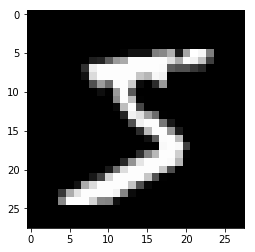Finally, let's create a dataloader to load the images in batches.

In :
``````from torch.utils.data import DataLoader

batch_size = 100
data_loader = DataLoader(mnist, batch_size, shuffle=True)``````

We'll also create a `device` which can be used to move the data and models to a GPU, if one is available.

In :
``````# Device configuration
device = torch.device('cuda' if torch.cuda.is_available() else 'cpu')
device
``````
Out:
``device(type='cuda')``

### Discriminator Network

The discriminator takes an image as input, and tries to classify it as "real" or "generated". In this sense, it's like any other neural network. While we can use a CNN for the discriminator, we'll use a simple feedforward network with 3 linear layers to keep things since. We'll treat each 28x28 image as a vector of size 784.

In :
``````image_size = 784
hidden_size = 256``````
In :
``````import torch.nn as nn

D = nn.Sequential(
nn.Linear(image_size, hidden_size),
nn.LeakyReLU(0.2),
nn.Linear(hidden_size, hidden_size),
nn.LeakyReLU(0.2),
nn.Linear(hidden_size, 1),
nn.Sigmoid())
``````

We use the Leaky ReLU activation for the discriminator.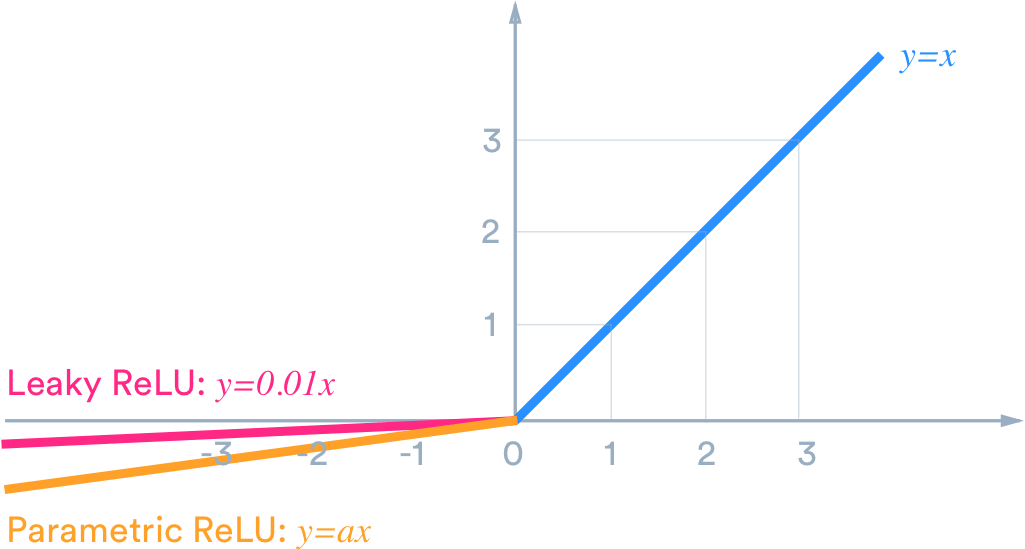Different from the regular ReLU function, Leaky ReLU allows the pass of a small gradient signal for negative values. As a result, it makes the gradients from the discriminator flows stronger into the generator. Instead of passing a gradient (slope) of 0 in the back-prop pass, it passes a small negative gradient. - Source

Just like any other binary classification model, the output of the discriminator is a single number between 0 and 1, which can be interpreted as the probability of the input image being fake i.e. generated.

Let's move the discriminator model to the chosen device.

In :
``D.to(device);``

### Generator Network

The input to the generator is typically a vector or a matrix which is used as a seed for generating an image. Once again, to keep things simple, we'll use a feedfoward neural network with 3 layers, and the output will be a vector of size 784, which can be transformed to a 28x28 px image.

In :
``latent_size = 64``
In :
``````G = nn.Sequential(
nn.Linear(latent_size, hidden_size),
nn.ReLU(),
nn.Linear(hidden_size, hidden_size),
nn.ReLU(),
nn.Linear(hidden_size, image_size),
nn.Tanh())
``````

We use the TanH activation function for the output layer of the generator."The ReLU activation (Nair & Hinton, 2010) is used in the generator with the exception of the output layer which uses the Tanh function. We observed that using a bounded activation allowed the model to learn more quickly to saturate and cover the color space of the training distribution. Within the discriminator we found the leaky rectified activation (Maas et al., 2013) (Xu et al., 2015) to work well, especially for higher resolution modeling." - Source

Note that since the outputs of the TanH activation lie in the range `[-1,1]`, we have applied the same transformation to the images in the training dataset. Let's generate an output vector using the generator and view it as an image by transforming and denormalizing the output.

In :
``````y = G(torch.randn(2, latent_size))
gen_imgs = denorm(y.reshape((-1, 28,28)).detach())``````
In :
``#gen_inp = torch.randn(2, latent_size)``
In :
``plt.imshow(gen_imgs, cmap='gray');``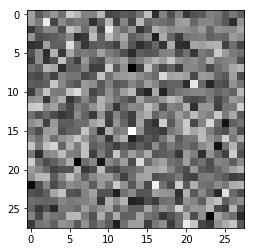In :
``plt.imshow(gen_imgs, cmap='gray');``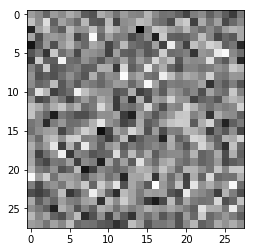As one might expect, the output from the generator is basically random noise. Let's define a helper function which can save a batch of outputs from the generator to a file.

Let's move the generator to the chosen device.

In :
``G.to(device);``

### Discriminator Training

Since the discriminator is a binary classification model, we can use the binary cross entropy loss function to quantify how well it is able to differentiate between real and generated images.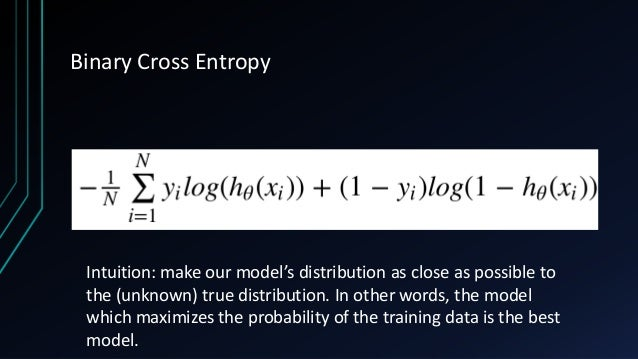In :
``````criterion = nn.BCELoss()
d_optimizer = torch.optim.Adam(D.parameters(), lr=0.0002)``````

Let's define helper functions to reset gradients and train the discriminator.

In :
``````def reset_grad():
d_optimizer.zero_grad()
g_optimizer.zero_grad()

def train_discriminator(images):
# Create the labels which are later used as input for the BCE loss
real_labels = torch.ones(batch_size, 1).to(device)
fake_labels = torch.zeros(batch_size, 1).to(device)

# Loss for real images
outputs = D(images)
d_loss_real = criterion(outputs, real_labels)
real_score = outputs

# Loss for fake images
z = torch.randn(batch_size, latent_size).to(device)
fake_images = G(z)
outputs = D(fake_images)
d_loss_fake = criterion(outputs, fake_labels)
fake_score = outputs

# Combine losses
d_loss = d_loss_real + d_loss_fake
# Reset gradients
reset_grad()
# Compute gradients
d_loss.backward()
# Adjust the parameters using backprop
d_optimizer.step()

return d_loss, real_score, fake_score``````

### Generator Training

Since the outputs of the generator are vectors (which can be transformed to images), it's not obvious how we can "train" the generator. This is where we employ a rather elegant "trick". Since we know that the output images are "generated" or "fake", we can pass them into the discriminator, and compare the output of the discriminator with the ground truth (i.e. all fake), and use that to calculate the loss for the generator.

In other words, we the disciminiator as a part of the loss function. Here's what this looks like in code.

In :
``g_optimizer = torch.optim.Adam(G.parameters(), lr=0.0002)``
In :
``````def train_generator():
# Generate fake images and calculate loss
z = torch.randn(batch_size, latent_size).to(device)
fake_images = G(z)
labels = torch.ones(batch_size, 1).to(device)
g_loss = criterion(D(fake_images), labels)

# Backprop and optimize
reset_grad()
g_loss.backward()
g_optimizer.step()
return g_loss, fake_images``````

### Training the Model

Let's create a directory where we can save intermediate outputs from the generator to visually inspect the progress of the model

In :
``````import os

sample_dir = 'samples'
if not os.path.exists(sample_dir):
os.makedirs(sample_dir)
``````

We are now ready to train the model. We train the discriminator first, and then the generator. The training might take a while if you're not using a GPU.

In :
``num_epochs = 20``
In :
``````from torchvision.utils import save_image

total_step = len(data_loader)
for epoch in range(num_epochs):
for i, (images, _) in enumerate(data_loader):
# Load a batch & transform to vectors
images = images.reshape(batch_size, -1).to(device)

# Train the discriminator and generator
d_loss, real_score, fake_score = train_discriminator(images)
g_loss, fake_images = train_generator()

# Inspect the losses
if (i+1) % 200 == 0:
print('Epoch [{}/{}], Step [{}/{}], d_loss: {:.4f}, g_loss: {:.4f}, D(x): {:.2f}, D(G(z)): {:.2f}'
.format(epoch, num_epochs, i+1, total_step, d_loss.item(), g_loss.item(),
real_score.mean().item(), fake_score.mean().item()))

# Save real images (just one batch)
if (epoch+1) == 1:
images = images.reshape(images.size(0), 1, 28, 28)
save_image(denorm(images), os.path.join(sample_dir, 'real_images.png'))

# Save sampled images
fake_images = fake_images.reshape(fake_images.size(0), 1, 28, 28)
fake_fname = 'fake_images-{}.png'.format(epoch+1)
print('Saving', fake_fname)
save_image(denorm(fake_images), os.path.join(sample_dir, fake_fname))
``````
```Epoch [0/20], Step [200/600], d_loss: 0.0591, g_loss: 4.1079, D(x): 0.99, D(G(z)): 0.05 Epoch [0/20], Step [400/600], d_loss: 0.1847, g_loss: 5.2257, D(x): 0.94, D(G(z)): 0.08 Epoch [0/20], Step [600/600], d_loss: 0.0716, g_loss: 5.3215, D(x): 0.97, D(G(z)): 0.03 Saving fake_images-1.png Epoch [1/20], Step [200/600], d_loss: 0.3236, g_loss: 3.5387, D(x): 0.89, D(G(z)): 0.15 Epoch [1/20], Step [400/600], d_loss: 0.1789, g_loss: 4.3792, D(x): 0.90, D(G(z)): 0.03 Epoch [1/20], Step [600/600], d_loss: 0.9233, g_loss: 4.7200, D(x): 0.79, D(G(z)): 0.31 Saving fake_images-2.png Epoch [2/20], Step [200/600], d_loss: 0.3192, g_loss: 3.7633, D(x): 0.89, D(G(z)): 0.08 Epoch [2/20], Step [400/600], d_loss: 0.7036, g_loss: 2.4899, D(x): 0.88, D(G(z)): 0.32 Epoch [2/20], Step [600/600], d_loss: 0.3913, g_loss: 3.6907, D(x): 0.84, D(G(z)): 0.09 Saving fake_images-3.png Epoch [3/20], Step [200/600], d_loss: 0.5829, g_loss: 2.5426, D(x): 0.89, D(G(z)): 0.31 Epoch [3/20], Step [400/600], d_loss: 0.7212, g_loss: 2.4872, D(x): 0.80, D(G(z)): 0.26 Epoch [3/20], Step [600/600], d_loss: 0.7519, g_loss: 2.2303, D(x): 0.87, D(G(z)): 0.37 Saving fake_images-4.png Epoch [4/20], Step [200/600], d_loss: 0.6518, g_loss: 2.9605, D(x): 0.84, D(G(z)): 0.21 Epoch [4/20], Step [400/600], d_loss: 0.4468, g_loss: 2.8051, D(x): 0.87, D(G(z)): 0.15 Epoch [4/20], Step [600/600], d_loss: 0.6607, g_loss: 2.0501, D(x): 0.84, D(G(z)): 0.23 Saving fake_images-5.png Epoch [5/20], Step [200/600], d_loss: 0.2544, g_loss: 3.4494, D(x): 0.90, D(G(z)): 0.09 Epoch [5/20], Step [400/600], d_loss: 0.5448, g_loss: 3.3782, D(x): 0.81, D(G(z)): 0.10 Epoch [5/20], Step [600/600], d_loss: 0.3795, g_loss: 2.8544, D(x): 0.88, D(G(z)): 0.08 Saving fake_images-6.png Epoch [6/20], Step [200/600], d_loss: 0.1991, g_loss: 3.4759, D(x): 0.92, D(G(z)): 0.07 Epoch [6/20], Step [400/600], d_loss: 0.4505, g_loss: 3.9452, D(x): 0.86, D(G(z)): 0.14 Epoch [6/20], Step [600/600], d_loss: 0.4136, g_loss: 3.5021, D(x): 0.90, D(G(z)): 0.17 Saving fake_images-7.png Epoch [7/20], Step [200/600], d_loss: 0.1741, g_loss: 4.1789, D(x): 0.93, D(G(z)): 0.06 Epoch [7/20], Step [400/600], d_loss: 0.3017, g_loss: 3.1273, D(x): 0.92, D(G(z)): 0.08 Epoch [7/20], Step [600/600], d_loss: 0.2238, g_loss: 4.6015, D(x): 0.93, D(G(z)): 0.07 Saving fake_images-8.png Epoch [8/20], Step [200/600], d_loss: 0.1162, g_loss: 4.4049, D(x): 0.96, D(G(z)): 0.05 Epoch [8/20], Step [400/600], d_loss: 0.2084, g_loss: 4.0611, D(x): 0.96, D(G(z)): 0.13 Epoch [8/20], Step [600/600], d_loss: 0.1736, g_loss: 3.6759, D(x): 0.96, D(G(z)): 0.10 Saving fake_images-9.png Epoch [9/20], Step [200/600], d_loss: 0.1049, g_loss: 6.7632, D(x): 0.97, D(G(z)): 0.06 Epoch [9/20], Step [400/600], d_loss: 0.1694, g_loss: 3.6628, D(x): 0.94, D(G(z)): 0.08 Epoch [9/20], Step [600/600], d_loss: 0.1861, g_loss: 4.0064, D(x): 0.97, D(G(z)): 0.12 Saving fake_images-10.png Epoch [10/20], Step [200/600], d_loss: 0.1043, g_loss: 3.6522, D(x): 0.98, D(G(z)): 0.06 Epoch [10/20], Step [400/600], d_loss: 0.1287, g_loss: 4.9207, D(x): 0.97, D(G(z)): 0.03 Epoch [10/20], Step [600/600], d_loss: 0.3523, g_loss: 4.2603, D(x): 0.91, D(G(z)): 0.05 Saving fake_images-11.png Epoch [11/20], Step [200/600], d_loss: 0.2389, g_loss: 4.8324, D(x): 0.98, D(G(z)): 0.17 Epoch [11/20], Step [400/600], d_loss: 0.3672, g_loss: 7.8075, D(x): 0.91, D(G(z)): 0.06 Epoch [11/20], Step [600/600], d_loss: 0.1951, g_loss: 4.8671, D(x): 0.95, D(G(z)): 0.04 Saving fake_images-12.png Epoch [12/20], Step [200/600], d_loss: 0.0883, g_loss: 5.8783, D(x): 0.97, D(G(z)): 0.02 Epoch [12/20], Step [400/600], d_loss: 0.3120, g_loss: 4.0738, D(x): 0.91, D(G(z)): 0.05 Epoch [12/20], Step [600/600], d_loss: 0.0494, g_loss: 8.2836, D(x): 0.97, D(G(z)): 0.01 Saving fake_images-13.png Epoch [13/20], Step [200/600], d_loss: 0.1101, g_loss: 6.6874, D(x): 0.96, D(G(z)): 0.01 Epoch [13/20], Step [400/600], d_loss: 0.1178, g_loss: 5.9751, D(x): 0.98, D(G(z)): 0.06 Epoch [13/20], Step [600/600], d_loss: 0.2790, g_loss: 5.3706, D(x): 0.91, D(G(z)): 0.07 Saving fake_images-14.png Epoch [14/20], Step [200/600], d_loss: 0.5231, g_loss: 3.4590, D(x): 0.93, D(G(z)): 0.23 Epoch [14/20], Step [400/600], d_loss: 0.4506, g_loss: 3.3489, D(x): 0.82, D(G(z)): 0.03 Epoch [14/20], Step [600/600], d_loss: 0.3607, g_loss: 3.6895, D(x): 0.92, D(G(z)): 0.05 Saving fake_images-15.png Epoch [15/20], Step [200/600], d_loss: 0.2346, g_loss: 5.2239, D(x): 0.94, D(G(z)): 0.06 Epoch [15/20], Step [400/600], d_loss: 0.2062, g_loss: 4.4178, D(x): 0.95, D(G(z)): 0.06 Epoch [15/20], Step [600/600], d_loss: 0.3168, g_loss: 4.0340, D(x): 0.94, D(G(z)): 0.06 Saving fake_images-16.png Epoch [16/20], Step [200/600], d_loss: 0.1823, g_loss: 8.3363, D(x): 0.93, D(G(z)): 0.01 Epoch [16/20], Step [400/600], d_loss: 0.1422, g_loss: 3.6265, D(x): 0.96, D(G(z)): 0.08 Epoch [16/20], Step [600/600], d_loss: 0.3754, g_loss: 3.3672, D(x): 0.89, D(G(z)): 0.12 Saving fake_images-17.png Epoch [17/20], Step [200/600], d_loss: 0.2513, g_loss: 4.4747, D(x): 0.97, D(G(z)): 0.14 Epoch [17/20], Step [400/600], d_loss: 0.3376, g_loss: 3.6248, D(x): 0.94, D(G(z)): 0.16 Epoch [17/20], Step [600/600], d_loss: 0.3894, g_loss: 3.6249, D(x): 0.88, D(G(z)): 0.08 Saving fake_images-18.png Epoch [18/20], Step [200/600], d_loss: 0.2150, g_loss: 4.9510, D(x): 0.93, D(G(z)): 0.03 Epoch [18/20], Step [400/600], d_loss: 0.3593, g_loss: 4.1883, D(x): 0.88, D(G(z)): 0.09 Epoch [18/20], Step [600/600], d_loss: 0.2693, g_loss: 4.4306, D(x): 0.97, D(G(z)): 0.15 Saving fake_images-19.png Epoch [19/20], Step [200/600], d_loss: 0.2201, g_loss: 4.1628, D(x): 0.96, D(G(z)): 0.12 Epoch [19/20], Step [400/600], d_loss: 0.2821, g_loss: 5.3919, D(x): 0.92, D(G(z)): 0.03 Epoch [19/20], Step [600/600], d_loss: 0.1595, g_loss: 4.8528, D(x): 0.96, D(G(z)): 0.07 Saving fake_images-20.png ```

Now that we have trained the models, we can save checkpoints.

In :
``````# Save the model checkpoints
torch.save(G.state_dict(), 'G.ckpt')
torch.save(D.state_dict(), 'D.ckpt')``````
In [ ]:
`` ``

### Save and Commit

In :
``pip install jovian --upgrade``
```Collecting jovian Downloading https://files.pythonhosted.org/packages/96/3c/472d7af5c9724ae4832537bbd3101d28247eabe4c1ce07cf147fcafa1093/jovian-0.1.89-py3-none-any.whl (42kB) |████████████████████████████████| 51kB 1.8MB/s eta 0:00:011 Collecting uuid Downloading https://files.pythonhosted.org/packages/ce/63/f42f5aa951ebf2c8dac81f77a8edcc1c218640a2a35a03b9ff2d4aa64c3d/uuid-1.30.tar.gz Requirement already satisfied, skipping upgrade: pyyaml in /opt/conda/lib/python3.6/site-packages (from jovian) (5.1.2) Requirement already satisfied, skipping upgrade: requests in /opt/conda/lib/python3.6/site-packages (from jovian) (2.22.0) Requirement already satisfied, skipping upgrade: chardet<3.1.0,>=3.0.2 in /opt/conda/lib/python3.6/site-packages (from requests->jovian) (3.0.4) Requirement already satisfied, skipping upgrade: urllib3!=1.25.0,!=1.25.1,<1.26,>=1.21.1 in /opt/conda/lib/python3.6/site-packages (from requests->jovian) (1.24.2) Requirement already satisfied, skipping upgrade: idna<2.9,>=2.5 in /opt/conda/lib/python3.6/site-packages (from requests->jovian) (2.8) Requirement already satisfied, skipping upgrade: certifi>=2017.4.17 in /opt/conda/lib/python3.6/site-packages (from requests->jovian) (2019.9.11) Building wheels for collected packages: uuid Building wheel for uuid (setup.py) ... done Created wheel for uuid: filename=uuid-1.30-cp36-none-any.whl size=6501 sha256=b3de8495886ee80880a4a7ee20129f68851f283417ffdb79652e55b496e68cca Stored in directory: /tmp/.cache/pip/wheels/2a/80/9b/015026567c29fdffe31d91edbe7ba1b17728db79194fca1f21 Successfully built uuid Installing collected packages: uuid, jovian Successfully installed jovian-0.1.89 uuid-1.30 Note: you may need to restart the kernel to use updated packages. ```
In :
``import jovian``
In :
``jovian.commit()``
```[jovian] Saving notebook.. ```
```[jovian] Creating a new notebook on https://jovian.ml/ [jovian] Please enter your API key ( from https://jovian.ml/ ): API Key:········ [jovian] Uploading notebook.. [jovian] Capturing environment.. [jovian] Committed successfully! https://jovian.ml/mohantysoumya/notebook-source-703d9 ```
In [ ]:
``````jovian.commit(artifacts = [
'G.ckpt',
'D.ckpt',
'fake_images-5.png',
'fake_images-10.png',
'fake_images-15.png',
'fake_images-20.png',

])
``````
```[jovian] Saving notebook.. ```